6.4.6 Solving TSP1

We shall now present a heuristic algorithm for TSP1 [CHRI 76]. The algorithm consists of three major steps, each of which, in turn, consists of the application of other well-known algorithms that we have already discussed. A fourth step, which usually offers further improvements in the solution, can be easily added to the procedure and will be described separately.

The basic three steps of our heuristic algorithm produce a tour that is guaranteed to be less than 50 percent longer than the optimal tour. Although this may not sound particularly exciting, it turns out that this is the best "worst-case performance" achieved by any efficient TSP algorithm devised so far.

Consider n points that must be traversed by a TSP1 tour (symmetric distances, complete connectivity, triangular inequality). Then we have:

Heuristic Algorithm for TSP1 (Algorithm 6.6)
 STEP 1 Find the minimum spanning tree that spans the n points. Call this minimum spanning tree T. STEP 2 Let no of the n nodes of T be odd-degree nodes (no is always an even number). Find a minimum-length pairwise matching of these no nodes, using a matching algorithm. Let the graph consisting of the links contained in the optimal pairwise matching be denoted as M. Create a graph H consisting of the union of M and T (H = M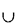T). Note that if one or more links are contained in both M and T. these links will appear twice in H. STEP 3 The graph H is an Eulerian graph, since it contains no odd-degree nodes. Draw an Eulerian circuit on H (beginning and ending at the starting node of the sought-after TSP tour, if such a starting node has already been specified). This Eulerian circuit is the (approximate) solution to the TSP.

Let us now pause to consider the characteristics and properties of the TSP solution whose derivation we just described. It should first be clear that the solution does indeed have the two properties of a traveling salesman tour that we specified earlier: namely, it is a circuit--beginning and ending at the same (specified or not) node--and it does visit each and every node at least once since it is an Eulerian circuit that covers all links of the Anode graph H that we have created.

What is now the relationship between the length of the tour that we have just obtained (i.e., the length of H) and the length of the actual optimum traveling salesman tour ? Although we have not found the optimum solution to the TSP, we can still place a bound on how far our solution can deviate from the optimum solution in the following way.

Let us denote by L(H), L(T), L(M), and L(TST) the length of H. T. and M, as defined, and of the (unknown) optimum traveling salesman tour, respectively.Proof: Suppose, for the moment, that somehow we have managed to obtain the optimum traveling salesman tour (TST). Since the TST is a circuit that covers all n points and visits each exactly once, it is clear that if we remove any one of the links on the TST, what will be left will be a spanning tree (not necessarily the minimum one) with n nodes and n - 1 links. Since T. by definition, is the minimum spanning tree, and since all distances are positive, it then follows that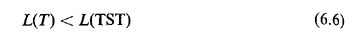In a similar vein, suppose that we took the TST and identified on it the n o points that were optimally matched in Step 2 of our algorithm. Suppose that we then matched pairwise, in an optimum manner, these no nodes by using only links that are contained in the TST. Let the set of all links used in this matching be denoted as M'. For the length of the subgraph M', L(M'), it must then be true that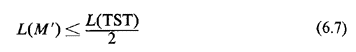For were this not so, M' could not possibly be the minimum length matching in TST of the n0 nodes.

At the same time, however, it is obvious that L(M)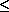L(M'), since m is a pairwise matching that is not restricted to the links that are contained in TST, and hence its length should be equal to or less than the length of M'. now combining (6.6) and (6.7) with the last statement and recognizing thatA fourth step, which can further improve the solution obtained at the end of Step 3, can also be added to Algorithm 6.6:
 STEP 4 (Optional): Check for nodes of H (points) that are visited more than once in the Eulerian tour and improve the traveling salesman tour of Step 3 by taking advantage of the triangular inequality.

For instance, if the tour at the conclusion of Step 3 reads, partly, as {A, B. C, D, B. E, . . .} where each capital letter represents a distinct point, we can consider improving the tour by using either the sequence {A, C, D, B. E, . . .} or {A, B. C, D, E, . . .}.

Note that Step 4 can be easily performed in O(n2) t--or even faster if one is careful. Since the three basic steps of Algorithm 6.6 also involve three efficient algorithms, the whole algorithm is efficient as well, and can be performed in O(n3) time. (Why?)

Finally, while Algorithm 6.6 can lead to solutions that are as much as 50 percent worse than the optimal, practical experience has shown that in applications involving tours with more than 50 or so points, the algorithm rarely gives solutions that are more than 10 percent worse than the optimal (provided that the points have not been prearranged so as to "defeat" the algorithm). In fact, the performance of Algorithm 6.6 can usually be expected to improve as the number of points, n, increases. On the other hand, it is possible to find "pathological" examples for which the worst-case performance is indeed attained asymptotically.

Exercise 6.6 Argue that for the arrangement of points shown in Figure 6.20, the solution given by the first three steps of Algorithm 6.6, approaches 3/2 · L (TST) as m increases. (Assume that e << 1.) note that, for m = 1, algorithm 6.6 gives the optimal tour.We now illustrate the application of Algorithm 6.6.
Example 9: Refuse-Collection Tour

The sanitation department of a small city faces the following problem. At nine distinct points in the city, solid refuse must be collected from the ends of dead-end alleys which are too narrow for regular-size garbage collection trucks. To deal with this situation the city has purchased a narrower vehicle which is now used exclusively for refuse collection at these nine special points.16The city wishes to find the shortest routefor the vehicle in question. Every day the vehicle must begin its tour at a depot and must return there--presumably to transfer its load to a regular-size truck--after visiting all nine collection points.

The location of the depot is shown as point 1 on Figure 6.21 and the nine collection points are numbered arbitrarily as points 2 through 10. We shall assume here that the actual distances between points can be well approximated by the Euclidean distances between them. These Euclidean distances for all pairs of points are shown, in turn, on Table 6-1.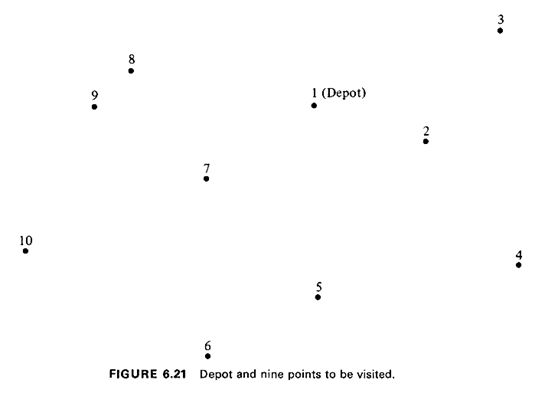Now applying our heuristic algorithm for the TSP, we first obtain, at the conclusion of Step 1, the minimum spanning tree, T (see Figure 6.22). The length of T is 258. (This already provides a lower bound on the length of the optimum traveling salesman tour.) There are six odd-degree nodes on T: 2, 3, 4, 6, 7, and 10. These nodes must be pairwise-matched in Step 2.

After completion of Step 2, the optimal pairwise matchings are 167, 2-3, and S4, for a total length of 143 units for the links in M. The graph H that results from the union of M and T is shown on Figure 6.23. The total length of H is 401 units, and one possible tour of this length is the tour {1, 2, 3, 2, 4, 6, 5, 7, 10, 9, 8, 7, 13.}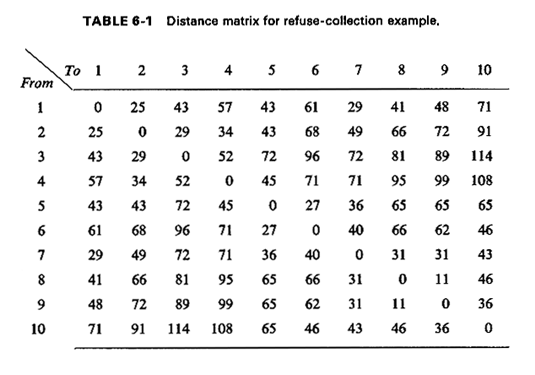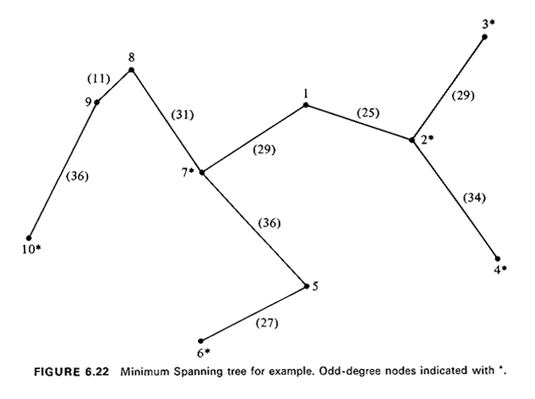Now applying Step 4 we note that nodes 2 and 7 are visited twice. The sequences {1, 3, 2, 4 . . .} and {1, 2, 3, 4, . . .} must then be compared withrespect to visiting node 2 and, similarly, the sequences {. . . , 5, 7, 10, 9, 8, 1} and {. . . , 5, 10, 9, 8, 7, 1} with respect to visiting node 7. A tie exists between the two former subsequences (we choose {1, 3, 2, 4, . . .} arbitrarily), whereas the subsequence {. . . , 5, 7,10, 9, 8,1} is the shorter of the two latter ones. Upon completion of Step 4 we thus obtain the tour {1, 3, 2, 4, 6, 5, 7, 10, 9, 8, 1} (shown in Figure 6.24), with a total length of 371 units. This we shall consider to be our "final" solution to the refusesollection problem.

The true optimal solution to this particular traveling salesman problem happens to be the tour { 1 , 3,2,4,5,6,10,9,8,7,1}, with a total length of 331 units. Thus, our solutions at the end of Step 3 and Step 4 were 21 percenT and 12 percent, respectively, inferior to the optimum (Figure 6.25).

It should also be clear that following completion of Step 4, one can often detect, by inspection, additional local permutations that lead to further improvements in the solution. For instance, from Figure 6.24 it is obvious that the subsequence {. . . , 4,5,6,7,...}is preferable to the one currently used, namely {. . . , 4,6,5,7,...}. Indeed, this change alone leads to a tour of length 347, or less than 5 percent longer than the optimum.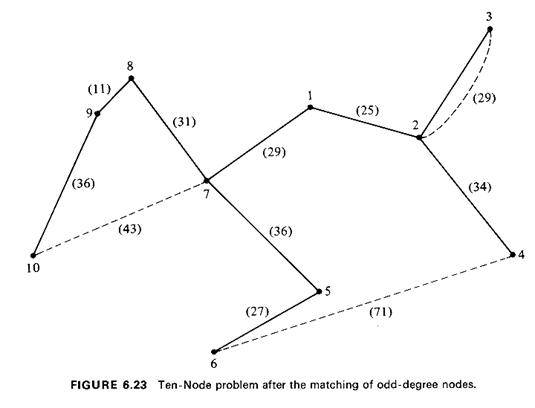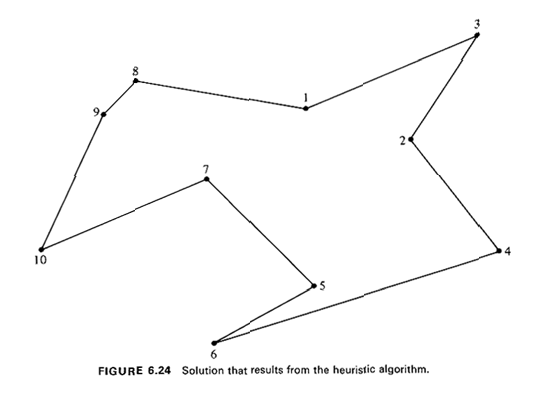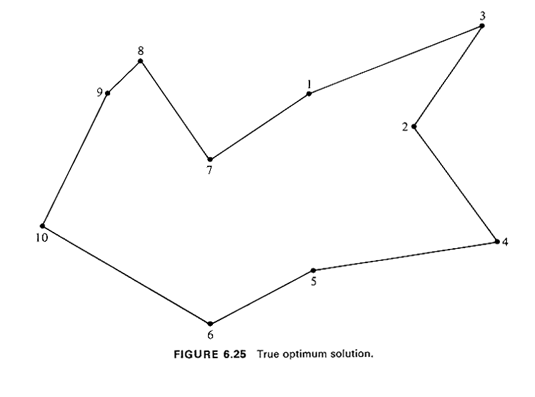16 This is a simplified version of a problem actually encountered in Brookline, Massachusetts by a colleague of the authors.